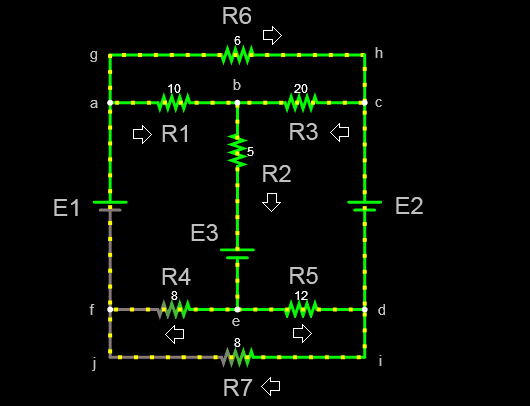# Can I solve this using Kirchoff's Law?

• Engineering
• Paul Lasdivan
The loop abcdefa is a combination of the other two loops, so it is not an independent equation. So we have 3 independent equations and 7 unknowns, which means there are infinitely many solutions. In summary, the given circuit has 7 resistors and 3 voltage sources. Using Kirchoff's laws, we can form 3 independent equations and 7 unknowns, leading to infinitely many solutions. This indicates that we may have overlooked an independent equation in the circuit.

#### Paul Lasdivan

Homework Statement
Can I solve this using Kirchoff's Law?
Relevant Equations
Kirchoff's LawWe were given a circuit with 7 resistors and 3 voltage sources
For emf sources, ##E_1=120V##, ##E_2=60V##, and ##E_3=30V## while for resistors in ohms,##R_1=10##, ##R_2=5##, ##R_3=20##, ##R_4=8##, ##R_5=12##, ##R_6=6##, ##R_7=8##. Nodes are indicated in small letters (a-j). Loops ##abefa##, ##abcdefa## and ##aghcdijfa## are assumed clockwise while the loop ##cbedc## is counterclockwise.

I applied Kirchoff's Current Rule in nodes ##b## and ##e##:

$$I_1+I_3 = I_2\\ I_4+I_5 = I_2$$

And then I apply the Voltage Rule:
Loop ##abefa##:
$$120-I_1R_1-I_2R_2-30-I_4R_4=0$$
Loop ##cbedc##:
$$60-I_3R_3-I_2R_2-30-I_5R_5=0$$
Loop ##abcdefa:##

$$120-I_1R_1+I_3R_3-60+I_5R_5-I_4R_4=0$$

Since I have 5 equations already with 5 unknows, hopefully I should solve these values.

From 1st and 2nd equations, ##I_4 = I_2-I_5 = I_1+I_3-I_5##. Setting ##I_4##, and ##I_2## in terms of ##I_1##, ##I_3## and ##I_5## only and substituting them to equations 3, 4 and 5, I get:

$$23I_1+13I_3-8I_5=90\\ 18I_1-12I_3-20I_5=60\\ 5I_1+25I_3+12I_5=30$$

Luckily my calculator supports systems of equations for 3 uknowns, but then I get a math error, which could indicate infinitely many solutions? Maybe I incorrectly applied Kirchoff's rule but I don't know where. Is it valid to apply Kirchoff's rule here?

I used MATLAB and got a solution from your equations. ##I_1=0.9256; I_3=2.8863; I_5=-3.8987##. The solution checks out. So your equations seem to be solvable.

The determinant of the matrix is very small, 7.7793e-13, so your guess of multiple solutions may be essentially correct. That may mean that you have overlooked an independent equation. I don't see one.

The abcdefa loop is just a combination of the other 2 loops, so you only have 2 equations with 3 unknowns. None of the loops involves R6 or R7 or the currents through them. I think you should end up with 4 equations and 4 unknowns

•FactChecker
Good catch. The loop aghcdijfa was listed but forgotten.

Paul Lasdivan said:
Homework Statement: Can I solve this using Kirchoff's Law?
Homework Equations: Kirchoff's Law

View attachment 248007

We were given a circuit with 7 resistors and 3 voltage sources
For emf sources, ##E_1=120V##, ##E_2=60V##, and ##E_3=30V## while for resistors in ohms,##R_1=10##, ##R_2=5##, ##R_3=20##, ##R_4=8##, ##R_5=12##, ##R_6=6##, ##R_7=8##. Nodes are indicated in small letters (a-j). Loops ##abefa##, ##abcdefa## and ##aghcdijfa## are assumed clockwise while the loop ##cbedc## is counterclockwise.

I applied Kirchoff's Current Rule in nodes ##b## and ##e##:

$$I_1+I_3 = I_2\\ I_4+I_5 = I_2$$

And then I apply the Voltage Rule:
Loop ##abefa##:
$$120-I_1R_1-I_2R_2-30-I_4R_4=0$$
Loop ##cbedc##:
$$60-I_3R_3-I_2R_2-30-I_5R_5=0$$
Loop ##abcdefa:##

$$120-I_1R_1+I_3R_3-60+I_5R_5-I_4R_4=0$$

Since I have 5 equations already with 5 unknows, hopefully I should solve these values.

From 1st and 2nd equations, ##I_4 = I_2-I_5 = I_1+I_3-I_5##. Setting ##I_4##, and ##I_2## in terms of ##I_1##, ##I_3## and ##I_5## only and substituting them to equations 3, 4 and 5, I get:

$$23I_1+13I_3-8I_5=90\\ 18I_1-12I_3-20I_5=60\\ 5I_1+25I_3+12I_5=30$$

Luckily my calculator supports systems of equations for 3 uknowns, but then I get a math error, which could indicate infinitely many solutions? Maybe I incorrectly applied Kirchoff's rule but I don't know where. Is it valid to apply Kirchoff's rule here?
It is ALWAYS correct to apply Kirchhoff's laws.
Even in electromagnetic circuits.
You just have to understand what "voltage" really is.

There are 7 unknown currents and 7 equations

3 loops:

aghcdijfa,abefa,cbedc

4 current enterings points:

b,f and a,d and c, and e